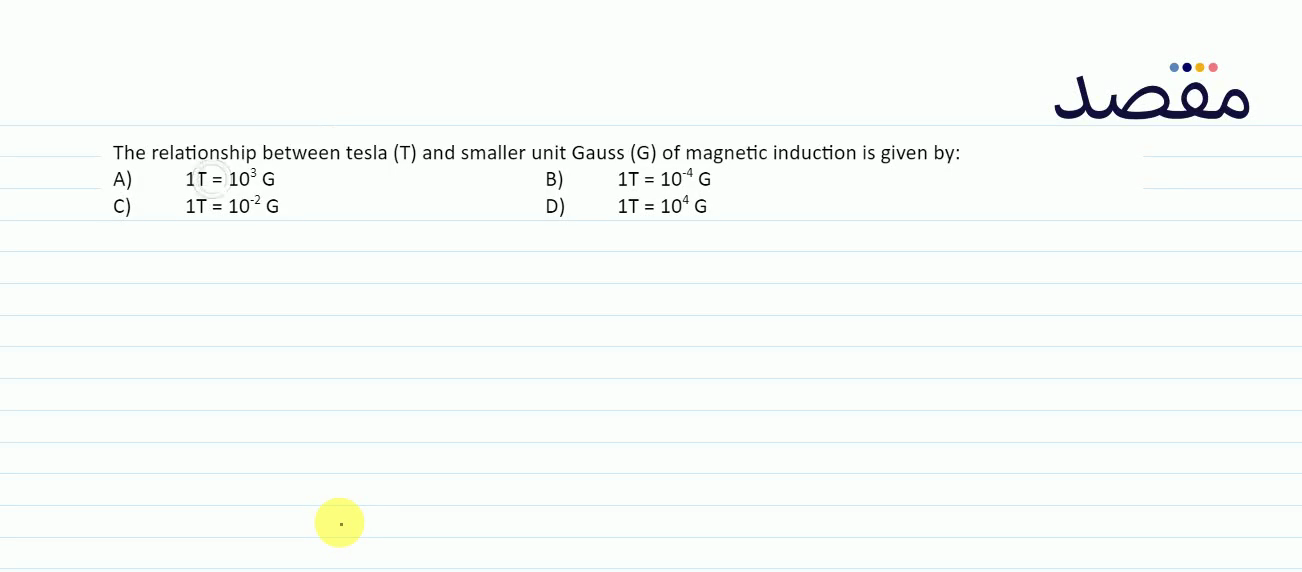# Classes

Change the way you learn with Maqsad's classes. Local examples, engaging animations, and instant video solutions keep you on your toes and make learning fun like never before!

Class 9Class 10First YearSecond Year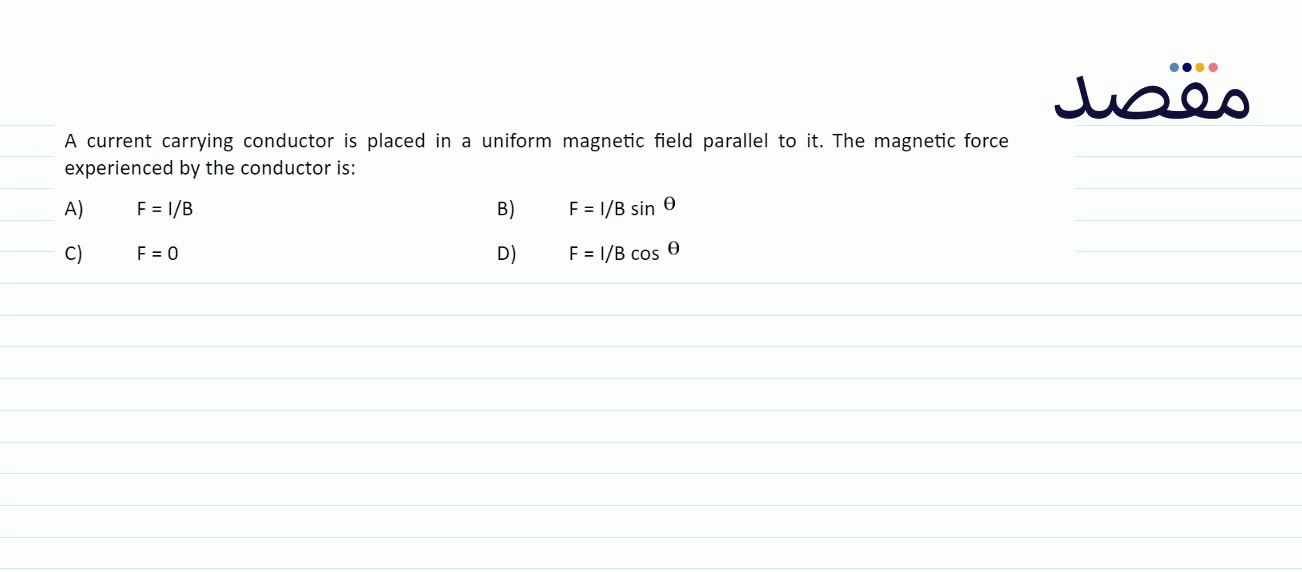### A current carrying conductor is placed in a uniform magnetic field parallel to it. The magnetic force experienced by the conductor is:A) F=I / B B) F=I / B \sin \theta C) \mathrm{F}=0 D) \mathrm{F}=\mathrm{l} / \mathrm{B} \cos \theta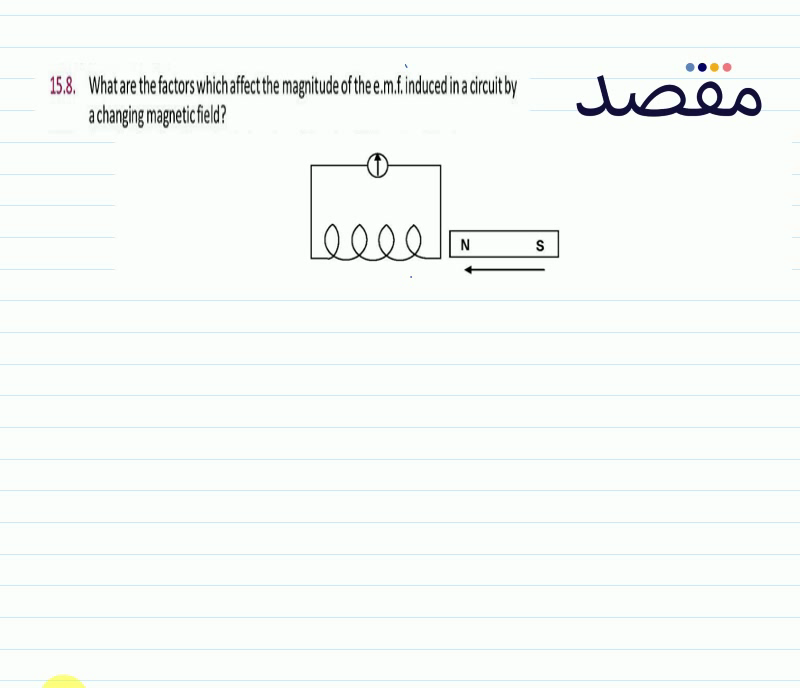### 15.8. What are the factors which affect the magnitude of the e.m.f. induced in a circuit by a changing magnetic field?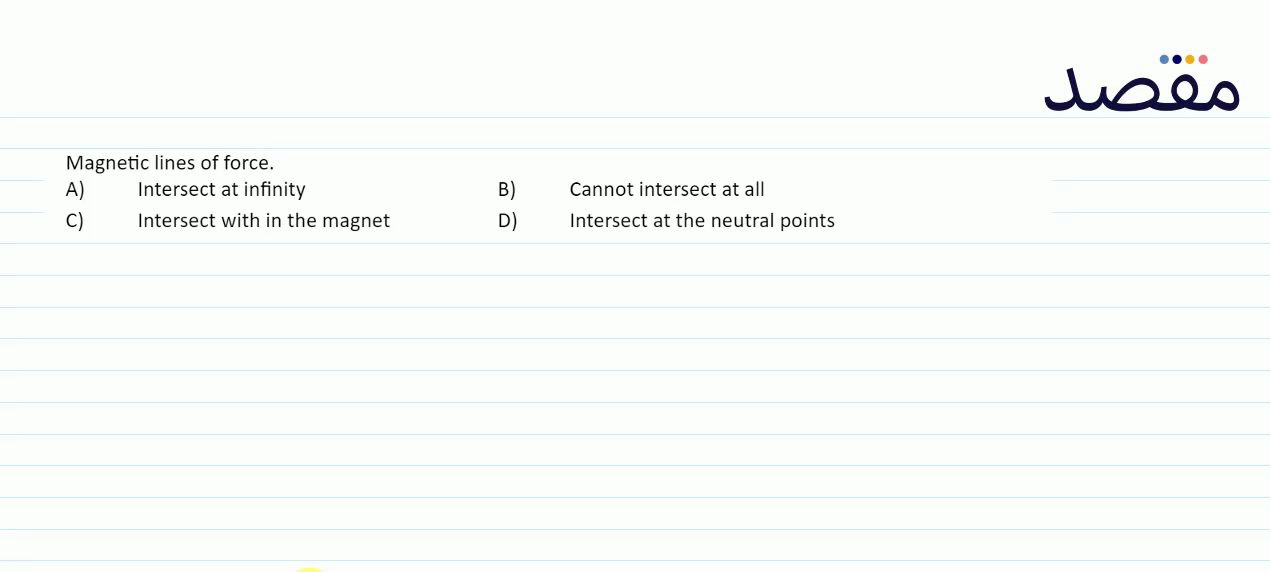### Magnetic lines of force.A) Intersect at infinityB) Cannot intersect at allC) Intersect with in the magnetD) Intersect at the neutral points### 14.12. An Iron core solenold with 400 turns has a cross section area of 4.0 \mathrm{~cm}^{2} . A current of 2 amp passing through it produces \mathrm{B}=0.5 webers-m { }^{-2} . How targe an emf is induced in it. If the current is twrned of in 0.1 seconds. What is the self Inductance of the solenold ? (Ans 0.8 \mathrm{~V} L=40 \mathrm{mh} ).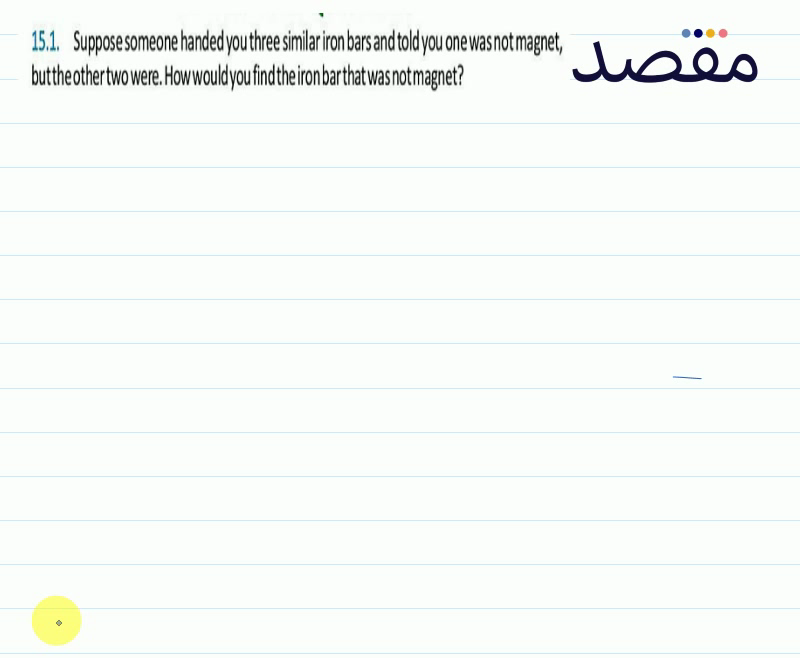### 15.1. Suppose someone handed you three similar iron bars and told you one was not magnet but the other two were. How would you find the iron bar that was notmagnet?